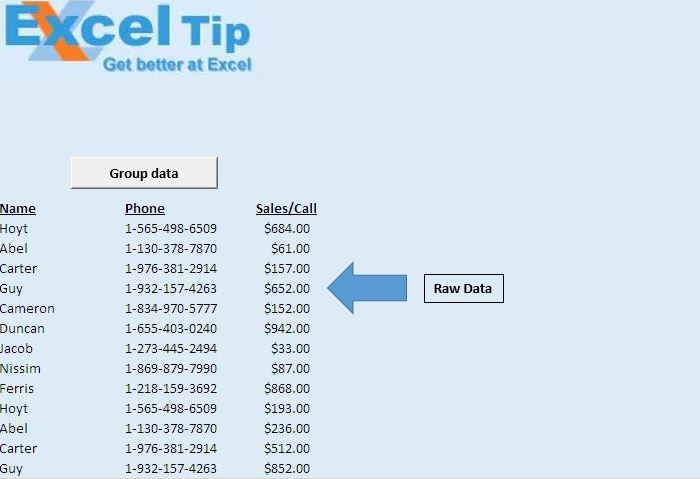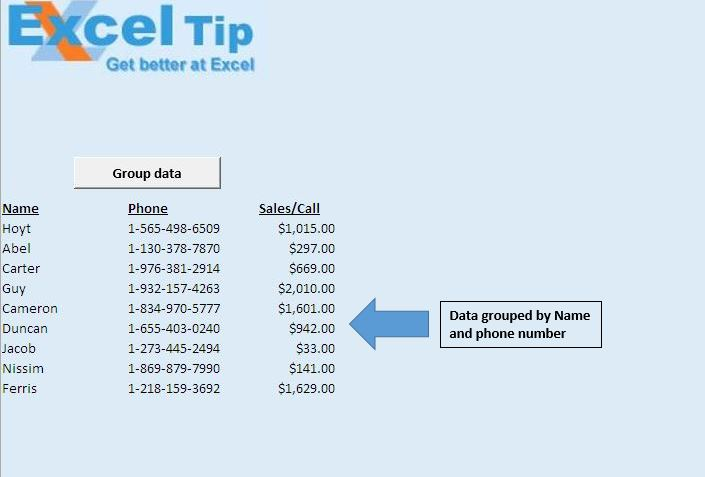# Grouping data using VBA in Microsoft Excel

In this article, we will create a macro to group data on behalf of certain conditions.

Raw data consists of Business Development team data. It consists of Name, Phone Number and Sales per call.In this article, we want to group data by agent name and the total revenue generated by each agent.Logic explanation

We have created “DataGrouping” macro, which performs the required calculation and grouping the data. It gives a unique agent name and the total revenue generated by each agent.

Code explanation

LngLastRow = Cells(Rows.Count, 1).End(xlUp).Row

The above code is used to get the row number of the last cell.

While Not Cells(i, 1).Value = ""

Wend

The above code is used to loop until a blank cell is encountered.

For LngRow = LngLastRow To (i + 1) Step -1

Next LngRow

The above For loop is used for reverse looping, starting from the last row to specify first row.

Rng.Offset(0, 2).Value = Rng.Offset(0, 2).Value + Cells(LngRow, 3).Value

The above code is used to sum up the values based on the specified criteria.

Rows(LngRow).Delete

The above code is used to delete the row.

```Option Explicit

Sub DataGrouping()

'Declaring variables
Dim Rng As Range
Dim LngRow As Long, LngLastRow, i As Long

Application.ScreenUpdating = False

'Getting row number of last cell
LngLastRow = Cells(Rows.Count, 1).End(xlUp).Row

'Initializing the first row
i = 12

'Looping until blank cell is encountered in first column
While Not Cells(i, 1).Value = ""

'Initializing range object
Set Rng = Cells(i, 1)

'Looping from last row to specified first row
For LngRow = LngLastRow To (i + 1) Step -1

'Checking whether value in the cell is equal to specified cell
If Cells(LngRow, 1).Value = Rng.Value Then
Rng.Offset(0, 2).Value = Rng.Offset(0, 2).Value + Cells(LngRow, 3).Value
Rows(LngRow).Delete
End If
Next LngRow

i = i + 1

Wend

Application.ScreenUpdating = True

End Sub
```

1.